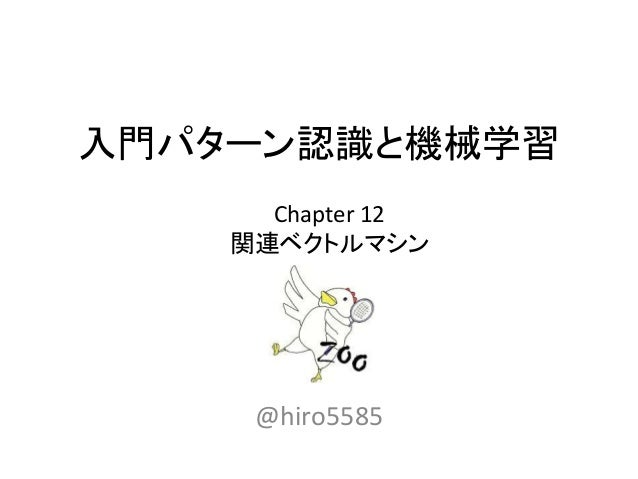Successfully reported this slideshow.Upcoming SlideShare
×

# 入門パターン認識と機械学習12章

1,315 views

Published on

Published in: Engineering
• Full Name
Comment goes here.

Are you sure you want to Yes No• Be the first to comment

### 入門パターン認識と機械学習12章

1. 1. 入門パターン認識と機械学習 @hiro5585 Chapter 12 関連ベクトルマシン
2. 2. 目次 １．RVMの概要 ２．グラフィカルモデルによるRVMの表現 ３．尤度と事前確率について ４．周辺化尤度の最大化 ５．更新式について ６．予測の方法について 2
3. 3. SVMとRVM サポートベクトルマシンも関連ベクトルマシンも識別境界を 引くための有効なベクトル（点）だけを保持することで 予測の際の計算量を小さくする 3 SVM RVM 確立時期：1963年 モデルのタイプ：識別関数 計算方法：マージン最大化 サポートベクターの数：中程度 計算量(予測時)：中程度 確立時期：2001年 モデルのタイプ：生成モデル 計算方法：尤度最大化 サポートベクターの数：少量 計算量(予測時)：少量
4. 4. RVMの全体の流れ １．予測したい値𝑦はカーネル回帰で表せる ２． 𝑦に対するカーネル回帰の式を確率で表現する ３．スパース化のため重み𝑎に対する事前確率を導入する ４．(周辺化)尤度を求めてそれが最大となる𝐴, 𝛽を求める 4 𝑦 = 𝒂 𝚻 𝐊 𝒙 + 𝜀 p(𝑦|𝑎, 𝑋, 𝐴, 𝛽) = Ν(𝑦 |𝒂 𝚻 𝐊 𝒙 , 𝛽−1 ) 𝑎に対するハイパーパラメータ 𝜀に対するハイパーパラメータ p(𝑎|𝐴) = Ν(𝑎 |0, 𝐴−1 ) 𝛼 𝑘 = 𝑠 𝑘 2 𝑞𝑘 2 − 𝑠 𝑘 ⋯ 𝑞𝑘 2 ≥ 𝑠 𝑘 ∞ ⋯ 𝑞𝑘 2 < 𝑠 𝑘 𝛽 = 𝑛 − 𝑖=0 𝑛 (1 − 𝛼𝑖Σ 𝑎 𝑖𝑖) |𝑦 − 𝐺𝜇 𝑎|2 ・・・12.14式 ・・・12.21式 ・・・12.17式 ・・・12.44式 ・・・12.61式
5. 5. 計算の流れ(1/2) １．同時確率を求める ２．重み𝑎を積分消去して𝑦の尤度を求める ３．周辺化尤度の式を求める 5 p(y, 𝑎|X, A, B) = Ν(y|𝑎K(X), 𝛽−1)×Ν(𝑎 |0, 𝐴−1) p y|X, A, 𝛽 = ∫ p(y, 𝑎 |X, A, β) d𝑎 = Ν(y|0, Σ)・・・12.32式 ・・・12.22式 L(A, β) = log|Σ| + 𝑦 𝑇Σ−1 𝑦 + (定数) ・・・12.34式
6. 6. 計算の流れ(2/2) ４．要素𝑎 𝑘ごとに微分をするため尤度をばらす ５． 12.43式で偏微分を行い𝑎 𝑘の更新式を得る ６．尤度の各項ごとをβで偏微分する(12.56, 12.59式) ７．結果をまとめて(12.60式)、βの更新式を得る 6 L(A, β) =log|Σ/𝑘| + log(1 + 𝑠 𝑘 𝛼 𝑘 ) + 𝑦 𝑇Σ/𝑘 −1 𝑦 − 𝑞 𝑘 2 𝛼 𝑘+𝑠 𝑘 ・・・12.40式 𝛼 𝑘 = 𝑠 𝑘 2 𝑞𝑘 2 − 𝑠 𝑘 ⋯ 𝑞𝑘 2 ≥ 𝑠 𝑘 ∞ ⋯ 𝑞𝑘 2 < 𝑠 𝑘 ・・・12.44式 𝛽 = 𝑛 − 𝑖=0 𝑛 (1 − 𝛼𝑖Σ 𝑎 𝑖𝑖 ) |𝑦 − 𝐺𝜇 𝑎|2 ・・・12.61式
7. 7. 目次 １．RVMの概要 ２．グラフィカルモデルによるRVMの表現 ３．尤度と事前確率について ４．周辺化尤度の最大化 ５．更新式について ６．予測の方法について 7
8. 8. グラフィカルモデル 確率変数間をグラフで表現したもの 可視化することで変数間の従属(独立)性が分かりやすくなる 8 a b c p(a, b, c) = p(a) x p(b|a) x p(c|a, b) a b c p(a, b, c) = p(b) x p(a|b) x p(c| b)
9. 9. RVMのグラフィカルモデル表現 重みのハイパーパラメータからカーネル関数の重みが生成 重み、データ、分散のハイパーパラメータから回帰値生成 9 a y X A β 重みを制御する ハイパーパラメータ データ 回帰値の分散を制御する ハイパーパラメータ カーネル関数の重み 回帰値 （クラス） RVMモデル 観測できるものは 黒く塗りつぶす
10. 10. RVMの確率的表現(1/2) 10 a y X A β 重みを制御する ハイパーパラメータ データ 回帰値の分散を制御する ハイパーパラメータ 回帰値 （クラス） RVMモデル p(a|X, y, A, β) = p(y, a|X, A, β) / p(y) ∝ p(y, a|X, A, β) = p(y|a, X, A, β) x p(a|A) 事後確率 尤度 事前確率 ベイズの定理 ・・・同時確率 事後確率と同時確率は 比例の関係を持つ カーネル関数の重み
11. 11. RVMの確率的表現(1/2) RVMでは尤度および事前確率にガウス分布を仮定する 事後確率を最大化するパラメータ(μとΣ)を求めればよい これをＭＡＰ(Maximum A Posteriori)推定という 11 p(a|X, y, A, β)∝p(y|a, X, A, β) x p(a|A) = Ν(y|aK(X), 𝛽−1 ) x Ν(a|0, 𝐴−1 ) = Ν(a|μ, Σ) 事後確率 尤度 事前確率
12. 12. 目次 １．RVMの概要 ２．グラフィカルモデルによるRVMの表現 ３．尤度と事前確率について ４．周辺化尤度の最大化 ５．更新式について ６．予測の方法について 12
13. 13. 尤度関数の意味(1/3) ここで、yがRVMではどのように表現されていたかを考える カーネル関数の重み付き和に誤差が加わったものが回帰値 グラム行列を用いて表現すると以下のようになる 13 𝑦 = 𝑗=0 𝑛 𝑎 𝑛 Κ 𝑥, 𝑥𝑗 + 𝜀 𝑦 = 𝒂 𝑻 𝐊 𝒙 + 𝜀
14. 14. 尤度関数の意味(2/3) 尤度と回帰式をもう一度眺めてみる ε はN(ε|0, 𝛽−1)から生起している白色雑音 14 p(y|a, X, A, B) = Ν(y|aK(X), 𝛽−1 ) 𝑦 = 𝒂 𝑻 𝐊 𝒙 + 𝜀
15. 15. 尤度関数の意味(3/3) aK(X)を中心に分散𝛽−1で散らばった分布からyは生起する 15 p(y|a, X, A, β) = Ν(y|aK(X), 𝛽−1 ) p(y) aK(X) 𝛽−1
16. 16. 事前確率の意味(1/3) 0を中心に分散𝛼−1の分布からaが生起する つまり、重みaは0付近の値を取る 16 p(a|α) = Ν(a|0, 𝛼−1 ) p(a|α) 0 𝛼−1
17. 17. 事前確率の意味(2/3) このとき、αが∞の場合を考えると𝛼−1が0となる 17 p(a|α) = Ν(a|0, 𝛼−1 ) p(a|α) 0 𝛼−1
18. 18. 事前確率の意味(3/3) 重みaが0になる確率が1となり対応するカーネルが無効化 事後確率最大化ではそのようなスパースなモデルを学習 18 p(a|α) = Ν(a|0, 0) p(a|α) 0 1.0 𝑦 = 𝑗=0 𝑛 𝑎 𝑛 Κ 𝑥, 𝑥𝑗 + 𝜀 元の式
19. 19. 目次 １．RVMの概要 ２．グラフィカルモデルによるRVMの表現 ３．尤度と事前確率について ４．周辺化尤度の最大化 ５．更新式について ６．予測の方法について 19
20. 20. エビデンス近似(1/2) しかしながら、事後確率のパラメータを求めるのは難しい そこで、方針を転換して事後確率に比例している 同時確率について計算をややこしくしてるaを積分消去する 20 p(a|X, y, A, β)∝Ν(a|μ, Σ) 事後確率 p(y, a|X, A, B) = p(y|a, X, A, β) x p(a|X, A, B) = p(y|a, X, B) x p(a|A) = Ν(y|aK(X), 𝛽−1 ) x Ν(a|0, 𝐴−1 ) 同時確率
21. 21. エビデンス近似(2/2) 同時確率について計算をややこしくしてるaを積分消去 そうすると、周辺化した尤度が出てくる 計算すると周辺化尤度はガウス分布になっている このように、パラメータを積分消去して周辺化尤度を求める ことをエビデンス近似という 周辺尤度を最大化するようなハイパーパラメータを求める 21 ∫ p(y, a|X, A, β) da = ∫ Ν(y|aK(X), 𝛽−1 ) x Ν(a|0, 𝐴−1 ) da = Ν(y|0, Σ) ∫ p(y, a|X, A, β) da = p(y|X, A, β) 周辺尤度
22. 22. 周辺化尤度の最大化 周辺尤度の対数をとり最大化を行う Aのチューニングは𝛼0, 𝛼1, ⋯ , 𝛼 𝑛を個別に偏微分して求める このとき、対象の𝛼𝑖以外は固定して求める 全ての𝛼𝑖が求まったら、𝛽で偏微分した値を使って𝛽を求める これを、収束条件に達するまで繰り返す 22 L(A, β) = log p(y|X, A, β) = log|Σ| + 𝑦 𝑇Σ−1 𝑦 + (定数) Σ = 𝐼 𝛽 + KA−1 𝐾 𝑇 A = 𝑑𝑖𝑎𝑔(𝛼0, 𝛼1, ⋯ , 𝛼 𝑛) チューニング対象
23. 23. 目次 １．RVMの概要 ２．グラフィカルモデルによるRVMの表現 ３．尤度と事前確率について ４．周辺化尤度の最大化 ５．更新式について ６．予測の方法について 23
24. 24. ハイパーパラメータの更新式 更新式は以下の通り 24 𝛼 𝑘 = 𝑠 𝑘 2 𝑞𝑘 2 − 𝑠 𝑘 ⋯ 𝑞𝑘 2 ≥ 𝑠 𝑘 ∞ ⋯ 𝑞𝑘 2 < 𝑠 𝑘 𝛽 = 𝑛 − 𝑖=0 𝑛 (1 − 𝛼𝑖Σ 𝑎 𝑖𝑖) |𝑦 − 𝐺𝜇 𝑎|2
25. 25. 目次 １．RVMの概要 ２．グラフィカルモデルによるRVMの表現 ３．尤度と事前確率について ４．周辺化尤度の最大化 ５．更新式について ６．予測の方法について 25
26. 26. RVMによる予測方法 新しいデータｘが入ってきたときの回帰値𝑦を求める 点推定で回帰値を予測する場合は期待値を用いる 26 p(y|𝑥, X, y, A, β) = ∫ p(y|𝑥, a, β)× p(a|X, y, A, β) da = Ν(a|𝜇 𝑎 𝑇 𝐤 𝑥 , 𝜎2(𝑥)) 𝑦 = 𝜇 𝑎 𝑇 𝐤 𝑥 12.25式